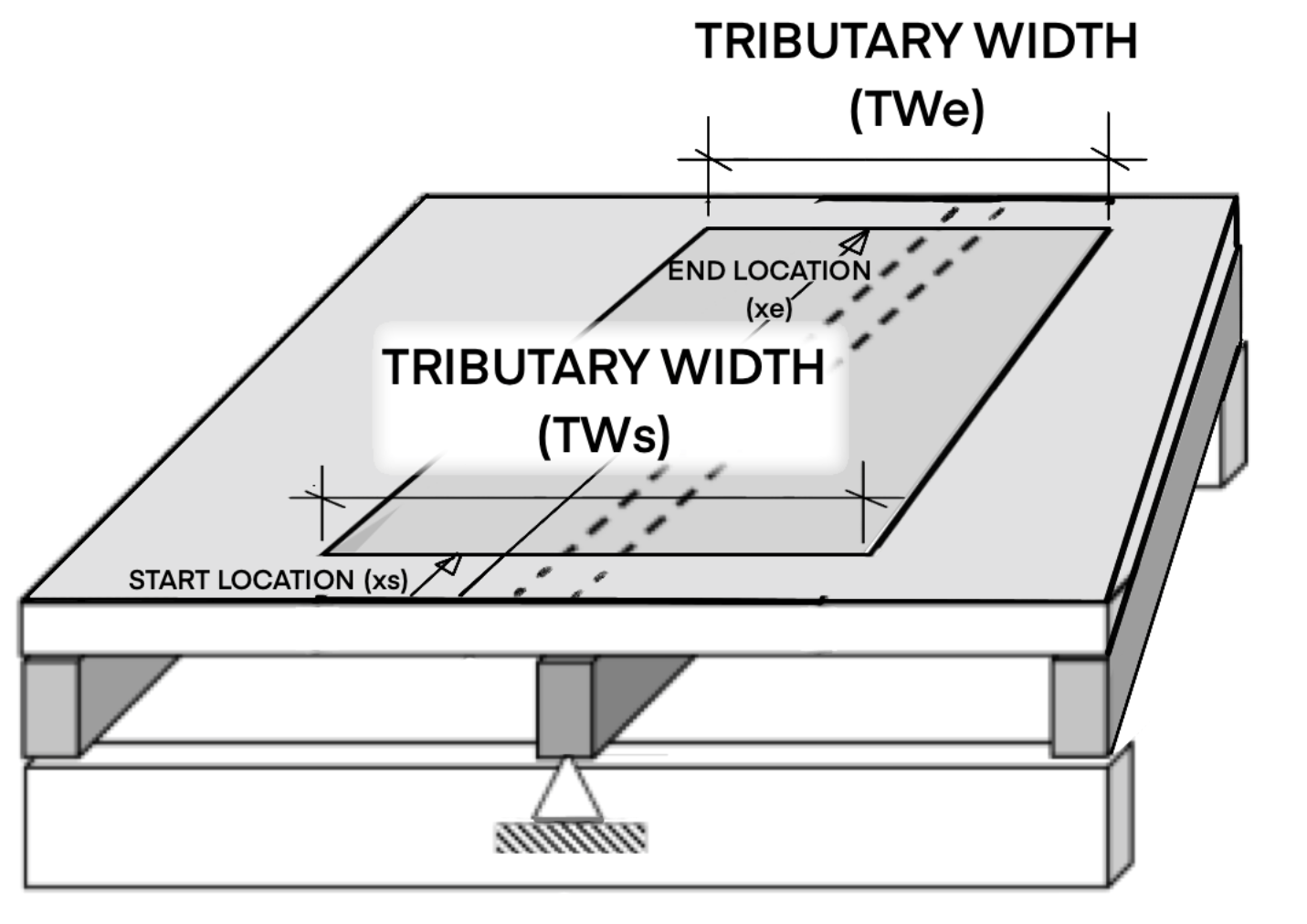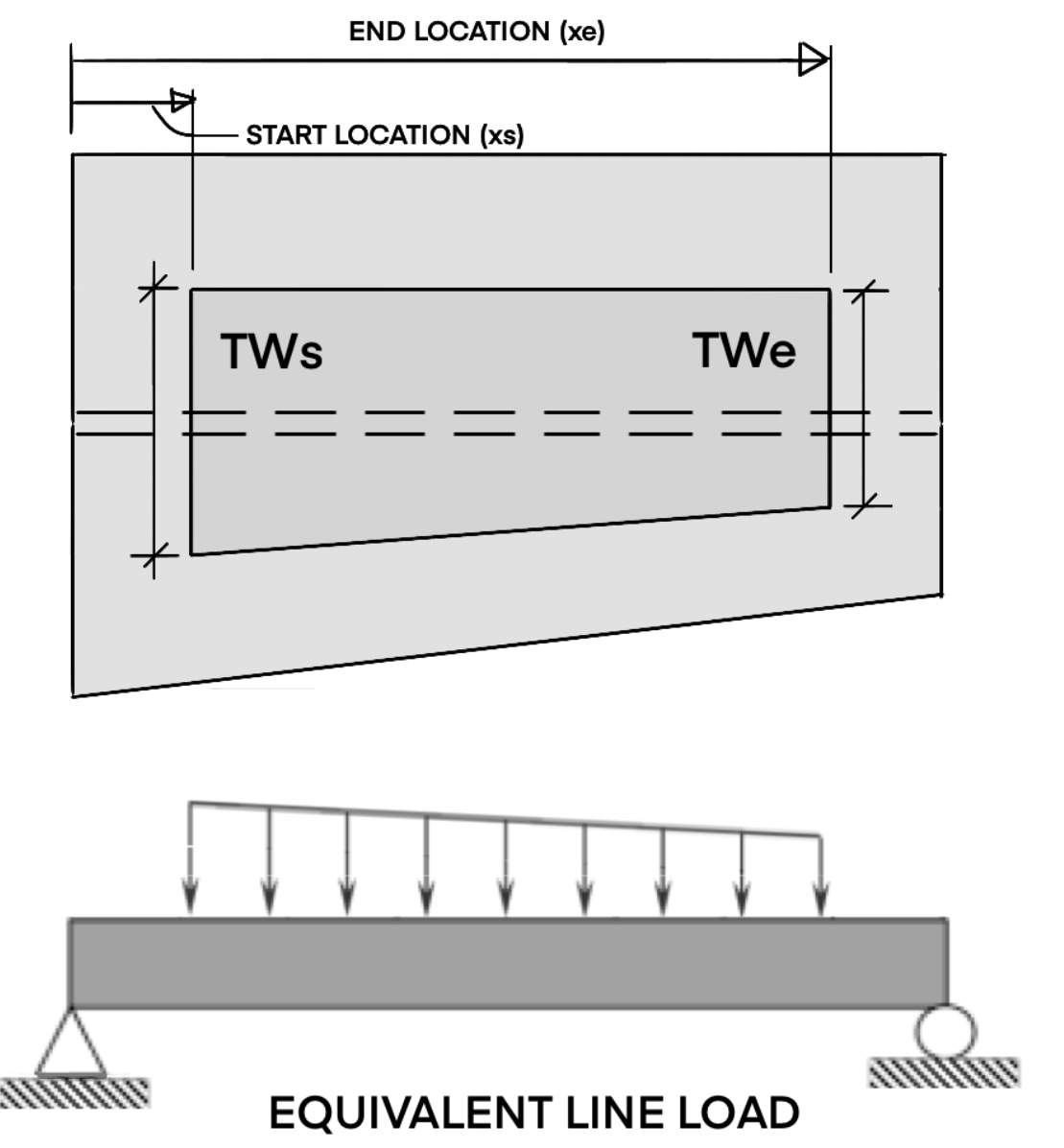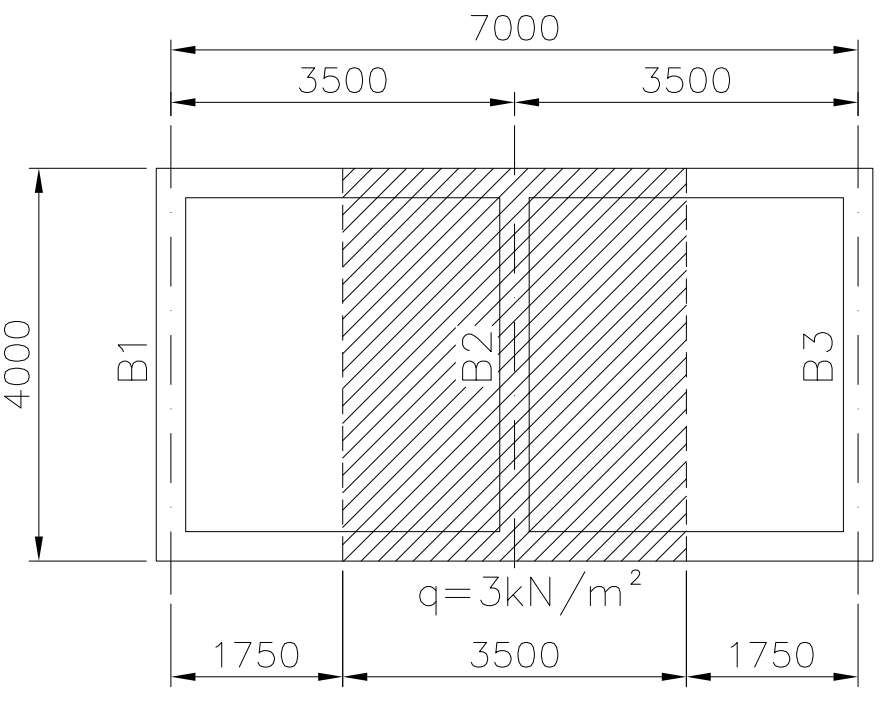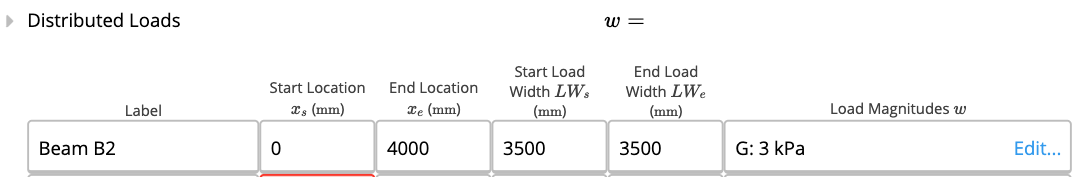# What is tributary width?

### 1. Background

Applying loading via a distributed / tributary area is useful when designing floors, roofs or other beams supporting slabs. The vertical or gravity loads are usually distributed one-way between regularly spaced joists or rafters, as depicted in Figure 2 below.

The load on a floor or a ceiling is distributed on the area of the structural element (force/area). The equivalent beam line load is equal to the "tributary width" multiplied by the "area load". E.g. 3 m (TWs/TWe) * 2.5 kPa (G/Q area load).

### 2. How to enter distributed loads in ClearCalcs

ClearCalcs provides an easy way to enter area loads by providing 4 dimensions and the area load.

• Start Location (x_s) - area loading may start at any point along the beam (e.g. only applied on one span)
• End Location (x_e)
• Start Load Width (TW_s) - may be different than TW_e as shown in Figure 2Figure 1 - ClearCalcs input box

ClearCalcs calculates the equivalent Line Load (see Figure 3) by multiplying the uniformly distributed load on the slab by the tributary width at each position along the beam.
E.g. if a load "q" in KPa (kN/m²) is applied on a slab and the tributary width "TW" is in meters length, the line load will be obtained by q x TW (kN/m).Figure 2 - 3D View of Load AreaFigure 3 - Plan and Elevation of Load Area

### 3. Worked Example

Given the structural arrangement in Figure 4, calculate the loads on the beams B1, B2, and B3. Consider a uniformly distributed load of 3 kPa.Figure 4 - Example Plan View

#### Solution:

Beam B2

L = 3kPa x 3.5m = 10.5 kN/m

Beam B1 = B3 (edge beam)

L = 3kPa x 3.5m / 2 = 5.25 kN/m

The tributary width is half of the spacing between the vertical beams.

When calculating the loads using ClearCalcs templates, the tributary width should be inputted into ClearCalcs as shown in Figure 5.Figure 5 - ClearCalcs input